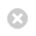Close

UCL Earth Sciences

Home# GEOL0018 Numerical Methods

This module covers the basic principles of numerical and computational methods to solve geophysical problems.

Coordinator: Dr Nicolas Brantut

Module details
TitleNumerical Methods
UG CodeGEOL0018
CoordinatorDr Nicolas Brantut
Other ContributorsDr Ana Fereirra
Term2
Credit15 credits
Written Exam
Coursework50% coursework and 50% mini project & presentation
Pre-RequisitesPHAS0002 Maths Method I, PHAS0009 Maths Method II or equivalent Maths modules with permission from the module organiser.

Basic knowledge of Matlab (or any equivalent language e.g. Python, Julia)
Maths & Stats Content and Requirement
Total Number of Hours of Student Work188 hours
Hours of Lectures/Seminars12 hours
Hours of Practicals/Problem Classes28 hours
Hours of Tutorials0
Days of Fieldwork0
OtherNone
Categorizing Student Performanve Levels

## GEOL0018GEOL0018

Content

The following topics will be introduced, discussed and analysed:

The module covers the basic principles of numerical and computational methods to solve geophysical problems. It is focused around the use of finite differences to solve differential equations. After an introduction to the concept of numerical solutions to the mathematical equations, the course will detail the concept of finite differences to solve initial value problems, boundary value problems and the heat equation. After the basic are covered, a series of applications will be presented covering a range of applied geophysical problems.

The module covers:

• Taylor expansions and concept of finite differences (FD);
• Solving ODEs using Euler and Runge Kutta methods;
• Solving BVPs using FD and iterative methods;
• Solving PDEs using explicit and implicit FD methods;
• Writing a report in LaTeX and representing data and model output;
• Solving the wave equation and using FD;
• Solving static mechanical equilibrium using FD and applications; Real time seismology and seismic hazard

#### AIMS

This aim of the module is to introduce standard computational methods to solve mathematical and geophysical problems such as heat diffusion, wave propagation and stress equilibrium.

#### OUTCOMES

By the end of the module students should:

• Understand how to discretsize and compute solutions to simplified differential equations;
• Understand the meaing and accuracy of the numerical solution and benchmarking techniques;
• Have a solid base of computational skills and be able to write their own codes and present their results from scratch using Matlab.